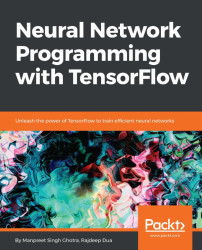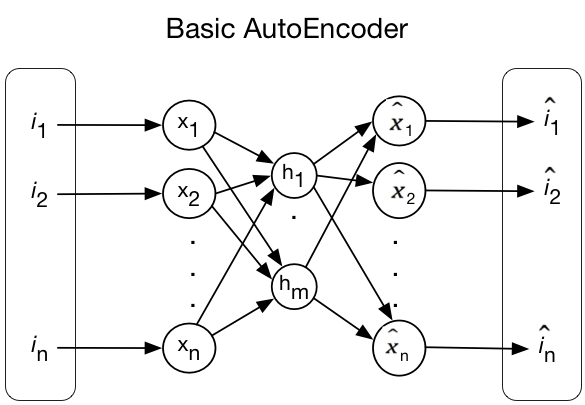•#### Neural Network Programming with Tensorflow#### Overview of this book

If you're aware of the buzz surrounding the terms such as "machine learning," "artificial intelligence," or "deep learning," you might know what neural networks are. Ever wondered how they help in solving complex computational problem efficiently, or how to train efficient neural networks? This book will teach you just that. You will start by getting a quick overview of the popular TensorFlow library and how it is used to train different neural networks. You will get a thorough understanding of the fundamentals and basic math for neural networks and why TensorFlow is a popular choice Then, you will proceed to implement a simple feed forward neural network. Next you will master optimization techniques and algorithms for neural networks using TensorFlow. Further, you will learn to implement some more complex types of neural networks such as convolutional neural networks, recurrent neural networks, and Deep Belief Networks. In the course of the book, you will be working on real-world datasets to get a hands-on understanding of neural network programming. You will also get to train generative models and will learn the applications of autoencoders. By the end of this book, you will have a fair understanding of how you can leverage the power of TensorFlow to train neural networks of varying complexities, without any hassle. While you are learning about various neural network implementations you will learn the underlying mathematics and linear algebra and how they map to the appropriate TensorFlow constructs.
Title PageCreditswww.PacktPub.comCustomer FeedbackPrefaceFree Chapter
Maths for Neural NetworksDeep Feedforward NetworksOptimization for Neural NetworksConvolutional Neural NetworksRecurrent Neural NetworksGenerative ModelsDeep Belief NetworkingAutoencodersResearch in Neural NetworksGetting started with TensorFlow## Chapter 8. Autoencoders

An autoencoder is a type of neural network that is trained to attempt to copy its input to its output. It has a hidden layer (let's call it h) that describes a code used to represent the input. The network may be viewed as consisting of two parts:

• Encoder function: h = f (x)
• Decoder that produces a reconstruction: r = g(h)

The following figure shows a basic autoencoder with input n and a hidden layer with neurons m:Basic representation of an autoencoder

Autoencoders are designed to be unable to learn to copy perfectly. They are restricted in ways that allow them to copy only approximately, and to copy only input that resembles the training data. As the model is forced to prioritize which aspects of the input should be copied, it often learns useful properties of the data.

The following topics will be covered in this chapter:

• Autoencoder algorithms
• Under-complete autoencoders
• Basic autoencoders